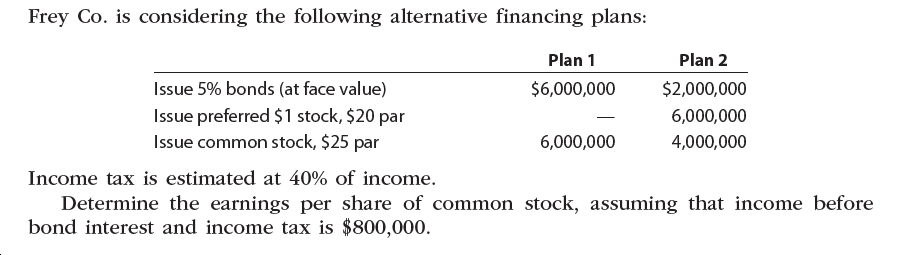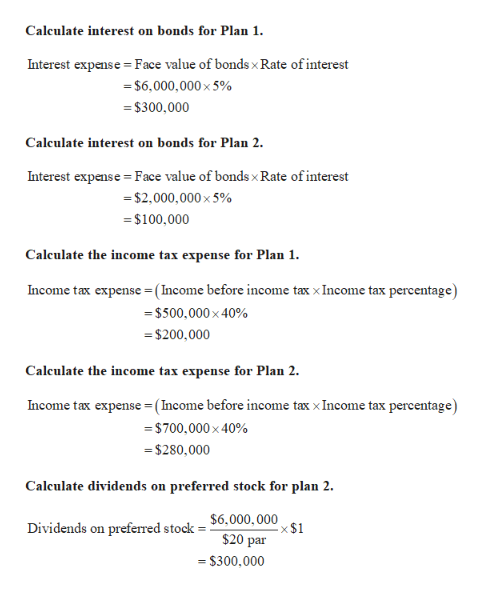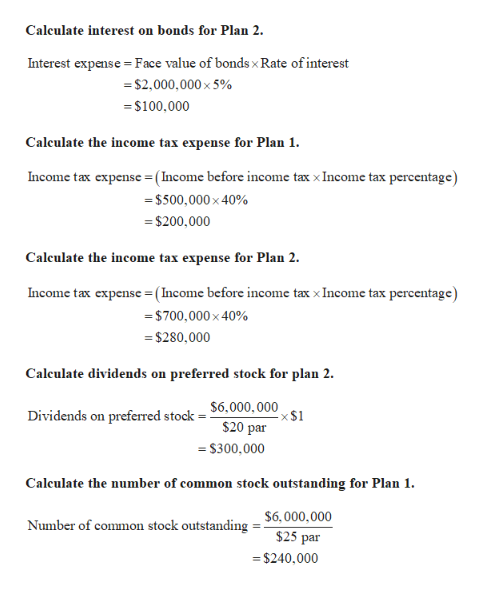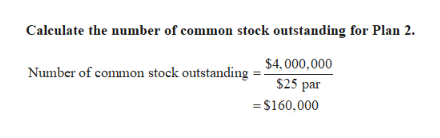# Frey Co. is considering the following alternative financing plans:Plan 1Plan 2Issue 5% bonds (at face value)\$6,000,000\$2,000,000Issue preferred \$1 stock, \$20 parIssue common stock, \$25 par6,000,0006,000,0004,000,000Income tax is estimated at 40% of income.Determine the earnings per share of common stock, assuming that income beforebond interest and income tax is \$800,000.

Question
1 viewshelp_outlineImage TranscriptioncloseFrey Co. is considering the following alternative financing plans: Plan 1 Plan 2 Issue 5% bonds (at face value) \$6,000,000 \$2,000,000 Issue preferred \$1 stock, \$20 par Issue common stock, \$25 par 6,000,000 6,000,000 4,000,000 Income tax is estimated at 40% of income. Determine the earnings per share of common stock, assuming that income before bond interest and income tax is \$800,000. fullscreen
check_circle

Step 1

Calculations:help_outlineImage TranscriptioncloseCalculate interest on bonds for Plan 1. Interest expense = Face value of bonds x Rate of interest = \$6,000,000 x 5% = \$300,000 Calculate interest on bonds for Plan 2. Interest expense = Face value of bonds x Rate of interest = \$2,000,000 x 5% = \$100,000 Calculate the income tax expense for Plan 1. Income tax expense = ( Income before income tax x Income tax percentage) = \$500,000 x 40% = \$200,000 Calculate the income tax expense for Plan 2. Income tx expense=(Income before income tax x Income tax percentage) = \$700,000 x 40% = \$280,000 Calculate dividends on preferred stock for plan 2. Dividends on preferred stock = \$6.000, 000 x \$1 \$20 par = \$300,000 fullscreen
Step 2help_outlineImage TranscriptioncloseCalculate interest on bonds for Plan 2. Interest expense = Face value of bonds x Rate of interest = \$2,000,000 x 5% = \$100,000 Calculate the income tax expense for Plan 1. Income tax expense=(Income before income tax x Income tax percentage) = \$500,000 x 40% = \$200,000 Calculate the income tax expense for Plan 2. Income tax expense = ( Income before income tax x Income tax percentage) = \$700,000 x 40% = \$280,000 Calculate dividends on preferred stock for plan 2. \$6,000, 000 ×\$1 Dividends on preferred stock \$20 par = \$300,000 Calculate the number of common stock outstanding for Plan 1. \$6,000,000 Number of common stock outstanding \$25 par = \$240,000 fullscreen
Step 3help_outlineImage TranscriptioncloseCalculate the number of common stock outstanding for Plan 2. \$4, 000,000 Number of common stock outstanding \$25 par = \$160,000 fullscreen

### Want to see the full answer?

See Solution

#### Want to see this answer and more?

Solutions are written by subject experts who are available 24/7. Questions are typically answered within 1 hour.*

See Solution
*Response times may vary by subject and question.
Tagged in